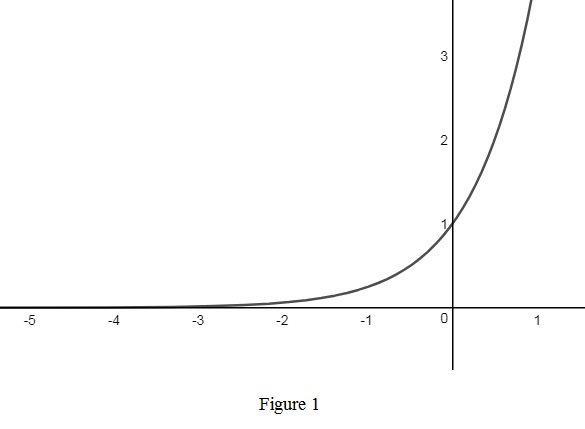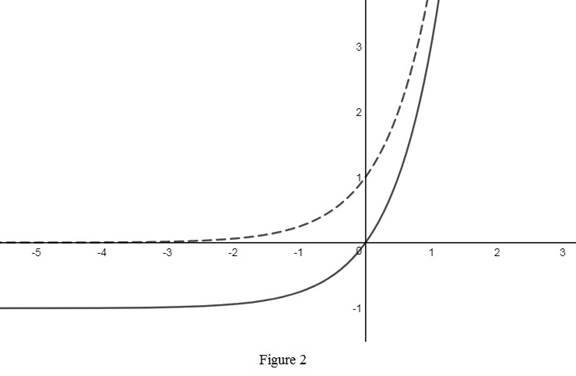# The rough graph of the function y = 4 x − 1 use transformations if needed.### Single Variable Calculus: Concepts...

4th Edition
James Stewart
Publisher: Cengage Learning
ISBN: 9781337687805### Single Variable Calculus: Concepts...

4th Edition
James Stewart
Publisher: Cengage Learning
ISBN: 9781337687805

#### Solutions

Chapter 1.5, Problem 11E
To determine

## To sketch: The rough graph of the function y=4x−1 use transformations if needed.

Expert Solution

### Explanation of Solution

The standard graph of the function y=4x is roughly sketched as shown below in Figure 1.Then, to draw the graph of y=4x1 , shift the graph y=4x one unit downward. Thus, the graph of y=4x1 is shown below in Figure 2.Observe that Figure 2 is obtained from Figure 1 in such a way that it is shifted 1 unit downward.

### Have a homework question?

Subscribe to bartleby learn! Ask subject matter experts 30 homework questions each month. Plus, you’ll have access to millions of step-by-step textbook answers!